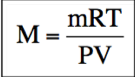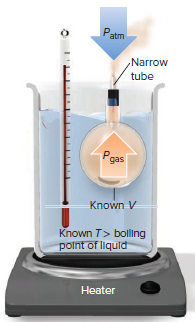# Problem: In the 19th century, J. B. A. Dumas devised a method for finding the molar mass of a volatile liquid from the volume, temperature, pressure, and mass of its vapor. He placed a sample of such a liquid in a flask that was closed with a stopper fitted with a narrow tube, immersed the flask in a hot water bath to vaporize the liquid, and then cooled the flask. Find the molar mass of a volatile liquid from the following:Mass of empty flask = 65.347 gMass of flask filled with water at 25°C = 327.4 gDensity of water at 25°C = 0.997 g/mLMass of flask plus condensed unknown liquid = 65.739 gBarometric pressure = 101.2 kPaTemperature of water bath = 99.8°C

###### FREE Expert Solution

Concept: Using Ideal Gas Law to find Molar Mass.

First make sure to find the mass of the volatile liquid and the volume of the flask (which equals to the volume of water at 25°C.

Then use this equation:90% (379 ratings)###### Problem Details

In the 19th century, J. B. A. Dumas devised a method for finding the molar mass of a volatile liquid from the volume, temperature, pressure, and mass of its vapor. He placed a sample of such a liquid in a flask that was closed with a stopper fitted with a narrow tube, immersed the flask in a hot water bath to vaporize the liquid, and then cooled the flask. Find the molar mass of a volatile liquid from the following:

Mass of empty flask = 65.347 g

Mass of flask filled with water at 25°C = 327.4 g

Density of water at 25°C = 0.997 g/mL

Mass of flask plus condensed unknown liquid = 65.739 g

Barometric pressure = 101.2 kPa

Temperature of water bath = 99.8°C